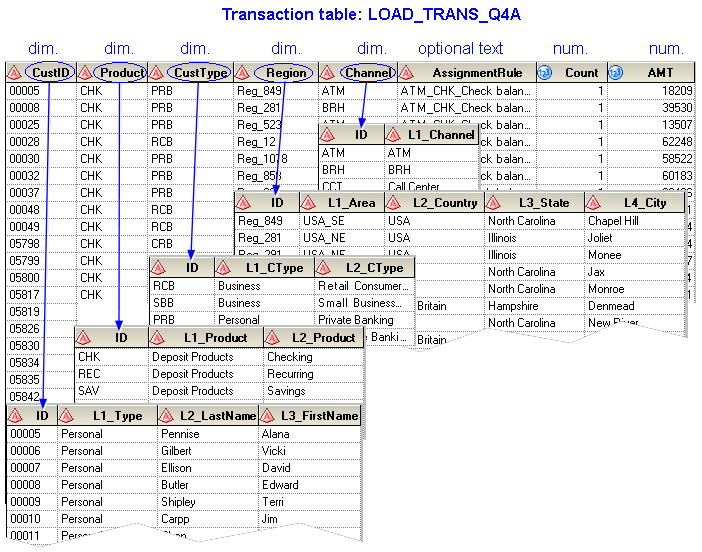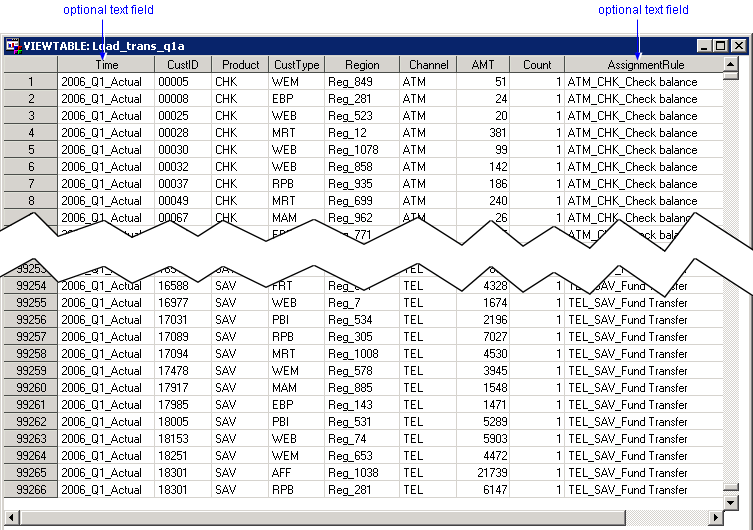Transaction Table

There must be one transaction table for each period in a model. For each table group, the transaction tables must have the same columns defined. A single model is likely to have multiple table groups. Multiple rules will likely use the same source table group. Transaction table layout is affected by the rule definition process (filter logic and driver logic). A transaction table contains the following columns and must conform to the following rules:

• Each column must have the field length shown.
• The position of columns is arbitrary.
• The name of the column is arbitrary.

Note: The following names, however, are reserved for use by Profitability Management as the names of columns created as a result of calculation:

Behavior
Quantity
Value
Period

• The number of columns in a transaction table is arbitrary.
• A transaction table can contain other columns not specifically used by Profitability Management.
 Name Maximum Field Length Description Dimensions (1 to n) Char 32 Each value identifies the row (in a custom dimensions table) for this transaction. The number of dimensions referred to by a transaction table is optional Driver Quantities (1 to n) Numeric 8 Numeric values that are used in a rule's driver formula to calculate the driver quantity for a transaction. The number of columns that are used to calculate the driver quantity for a transaction is optional. Optional columns (0 to n) optional You can add any number of optional text or numeric columns of any length to a transaction table. For example, you can use an optional column for matching fields in a behavior table.

The dimensions of a transaction are specified in custom dimensions tables. The following picture shows the mapping from a sample transaction table to different custom dimensions tables.

Note: When transaction tables in different transaction table groups map to the same custom dimensions table, the column names in the transaction tables must match. Otherwise, the table joins to create the OLAP cube will fail.The following is a sample transaction table: